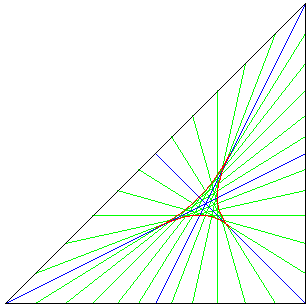Since you are reading this, you are not seeing the applet. If you are lucky, here is a picture:This java applet draws the medians and other area bisectors of a triangle. Click and drag within the applet area to change the triangle. What proportion of the triangle is the red deltoid? More information below.

# Medians and Area Bisectors of a Triangle

The blue lines above are the medians of the triangle. Each one connects a vertex of the triangle to the mid-point of the opposite edge. Each median divides the area of the triangle in half. They intersect two-thirds of the way along their length in the centroid of the triangle. The centroid is the centre of mass (or center of gravity - depending on your spelling and approach) of the solid triangle, so a solid triangle would balance on any line through the centroid.

However, it is not true that all straight lines through the centroid divide the area of the triangle in half. While the medians do divide the triangle into two equal areas, other lines through the centroid do not, and in the worst case (when the line through the centroid is parallel to an edge) the tringle on one side is 4/9 of the area of the original triangle, while the trapezium on the other side has an area 5/9 of the original triangle.

Some of the other lines which are area bisectors are shown above in green. Together, their envelope is a deltoid, shown in red, and any point inside the deltoid has three area bisectors passing through it, while any point outside it has only one. The curved edges of the detoid are segments of hyperbolae, and its three vertices are the mid-points of the medians. Since the proportions in the diagram are invariant under affine transformations, the deltoid's area is a fixed proportion of the area of the triangle. By taking a simple triangle, such as the one with corners at (0,0), (0,1) and (1,0), it is not difficult to find that the proportion is 3/4*loge(2)-1/2 = 0.019860... If this seems small (less than a fiftieth the original triangle), remember that the area of the triangle with straight edges using the corners of the deltoid is only a sixteenth of the area of the original triangle and the deltoid is almost a third of this smaller triangle.

It is easy to construct a set of points met by all the medians and other area bisectors. Indeed the intersection of the deltoid with a median or another area biscetor is such a set. The intersection of the deltoid and a median or other area bisector is 1/sqrt(2) - 1/2 = 0.2071... of the length of that median or area bisector.

See the Java code.

This page is based on discussions in the newsgroup geometry.puzzles in October and December 2001. Archives of these can be found here: 1 2 3 4 5.

Zak Seidov produced a couple of related pages on a 3,4, 5 triangle: Centroid and 3-points Deltoid Area

Rouben Rostamian produced a picture of the area of the deltoid

The following links to some of my pages are less directly relevant:

Henry Bottomley January 2002